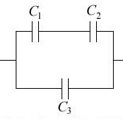# Three capacitors are connected as shown in the figure below. What is the equivalent capacitance?

Three capacitors are connected as shown in the figure below. What is the equivalent capacitance? (Take C1 =5.4 μF , C2 =15 μF ,and C3 =3.4 μF…)